# GSEB Solutions Class 7 Maths Chapter 6 The Triangles and Its Properties InText Questions

Gujarat Board GSEB Textbook Solutions Class 7 Maths Chapter 6 The Triangles and Its Properties InText Questions and Answers.

## Gujarat Board Textbook Solutions Class 7 Maths Chapter 6 The Triangles and Its Properties InText QuestionsTry These (Page 113)

Question 1.
Write the six elements (i.e. the 3 sides and the 3 angles) of ΔABC.
Solution:
Six elements of ∆ABC are: ∠A, ∠B, ∠C,
$$\overline{A B}$$, $$\overline{B C}$$ and $$\overline{C A}$$.

Question 2.
Write the:
(i) Side opposite to the vertex Q of ∆PQR
(ii) Angle opposite to the side LM of ∆LMN
(iii) Vertex opposite to the side RT of ∆RST
Solution:
(i) The Side opposite to the vertex Q of $$\overline{P R }$$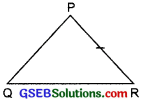(ii) The Angle opposite to the side LM of ∠N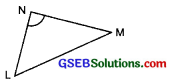(iii) Vertex opposite to the side RT is ‘S’ .Question 3.
Look at figures and classify each of the triangles according to its –
(a) Sides
(b) Angles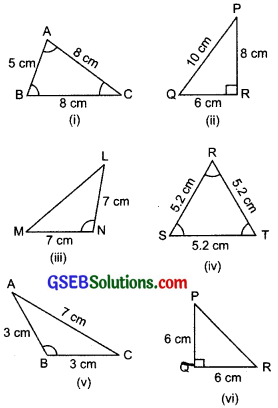Solution:
(i) (a) ∵ $$\overline{AC}$$ = $$\overline{B C }$$ = 8 cm
∴ ∆ABC is an isosceles triangle.

(b) Since all angles of ∆ABC are less than 90°
∴ It is an acute triangle.

(ii) (a) Since PQ ≠ QR ≠ RP
∴ ∆PQR is a scalene triangle.

(b) Since ∠R = 90°
∴ ∆PQR is a right triangle.

(iii) (a) In ∆LMN, LN = MN = 7 cm
∴ ∆LMN is an isosceles triangle.

(b) In ∆LMN, ∠N > 90°
∴ ∆LMN is an obtuse triangle.

(iv) (a) In ∆RST, RS = ST = TR = 5.2 cm
∴ It is an equilateral triangle.

(b) All the angles of ∆RST are acute.
∴ It is an acute triangle.

(v) (a) In ∆ABC, $$\overline{A B}$$ = $$\overline{B C}$$ = 3 cm
∴ It is an isosceles triangle.

(b) In ∆ABC, ∠B > 90°
∴ It is an obtuse triangle

(vi) (a) In ∆PQR, $$\overline{P Q}$$ = $$\overline{Q R}$$ = 6 cm
∴ It is an isosceles triangle.

(b) In ∆PQR, ∠Q = 90°
∴ It is a right triangle.Think, Discuss and Write (Page 114)

Question 1.
How many medians can a triangle have?
Solution:
Since a triangle has three sides and by joining the mid-point of each side to the opposite vertex, we can draw three line segments. Thus, a triangle can have three medians.

Question 2.
Does a median lie wholly in the interior of the triangle? (If you think that this is not true, draw a figure to show such a case.)
Solution:
Yes, a median lies wholly in the interior of the triangle.

Think, Discuss and Write (Page 115)

Question 1.
How many altitudes can a triangle have?
Solution:
An altitude can be drawn from each vertex of a triangle.
∴ A triangle can have three altitudes.Question 2.
Draw rough sketches of altitudes from A to $$\overline{B C}$$ for the following triangles.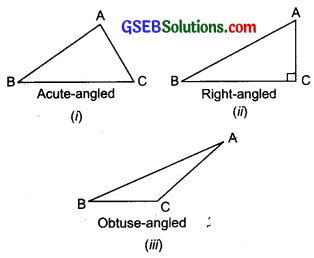Solution:
We have $$\overline{A L}$$ as an altitude in each case as shown below:Question 3.
Will an altitude always lie in the interior of a triangle? If you think that this need not be true, draw a rough sketch to show such a case.
Solution:
No, the altitude does not always lie in the interior of a triangle.
Example: In the adjoining figure, the altitude AD is not in the interior of ΔABC.Question 4.
Can you think of a triangle in which two altitudes of the triangle are two of its sides?
Solution:
Yes, a right triangle has two of its sides as its altitudes. In a right triangle ABC, $$\overline{A C}$$ and $$\overline{B C}$$ are its altitudes.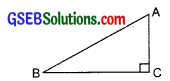Question 5.
Can the altitude and median be same for a triangle?
Solution:
Yes, in a triangle, its A median and altitude can be same line segment. In the adjoining figure, AL is an altitude as well as a median of ∆ABC.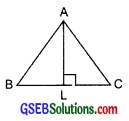Think, Discuss and Write (Page 117)

Question 1.
Exterior angles can be formed for a triangle in many ways. Three of them are shown here.There are three more ways of getting exterior angles. Try to produce those rough sketches.
Solution:
Three other exterior angles can be as under: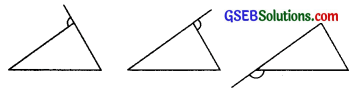Question 2.
Are the exterior angles formed at each vertex of a triangle equal?
Solution:
No.

Question 3.
What can you say about the sum of an exterior angle of a triangle and its adjacent interior angle?
Solution:
The sum of an exterior angle and its adjacent interior angle form a linear pair.
∴ [Exterior angle] + [Interior adjacent angle] = 180°.

Think, Discuss and Write (Page 118)

Question 1.
What can you say about each of the interior opposite angles, when the exterior angle is –
(i) a right angle?
(ii) an obtuse angle?
(iii) an acute angle?
Solution:
(i) Each of the interior angle is an acute angle.
(ii) At least one of the interior opposite angle must be an acute angle.
(iii) Each of the interior angles is an acute angle.

Question 2.
Can the exterior angle of a triangle be a straight angle?
Solution:
No.Try These (Page 118)

Question 1.
An exterior angle of a triangle is of measure 70° and one of its interior opposite angles is of measure 25°. Find the measure of the other interior opposite angle.
Solution: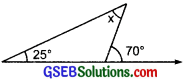Exterior angle = 70°
Interior opposite angles are 25° and x.
∴ x + 25° = 70°
[Using the exterior angle property of a triangle]
or x = 70° – 25° = 45°
∴ The required interior opposite angle = 45°.

Question 2.
The two interior opposite angles of an exterior angle of triangle are 60° and 80°. Find the measure of the exterior angle.
Solution:
Interior angles are 60° and 80°.
∵ [Exterior angle] = [Sum of the interior opposite angles]
∴ [Exterior angle] = 60° + 80° = 140°Question 3.
Is something wrong in this diagram? Comment.
Solution:
We know that an exterior angle of a triangle is equal to the sum of interior opposite angles.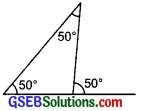Here interior angles are 50° angle is 50°.
∴ This triangle cannot be formed. [∵ 50° ≠ 50° + 50°]

Try These (Page 122)

Question 1.
Two angles of a triangle are 30° and 80°. Find the third angle.
Solution:
Let the third angle be x.
∴ Using the angle sum property of a triangle, we have
30° + 80° + x = 180°
or x + 110° = 180°
or x = 180° – 110° = 70°
∴ The measure of the required third angle is 70°.

Question 2.
One of the angles of a triangle is 80° and the other two angles are equal. Find the measure of each of the equal angles.
Solution:
Let each of the equal angles be x.
Using the angle sum property of a triangle, we have
x + x + 80° = 180°
or 2x + 80° = 180°
or 2x = 180° – 80° = 100°
or $$\frac { 2x }{ 2 }$$ = $$\frac { 100° }{ 2 }$$ or x = 50°
∴ The required measure of each of the equal angles is 50°.Question 3.
The three angles of a triangle are in the ratio 1:2:1. Find all the angles of the triangle. Classify the triangle in two different ways.
Solution:
Let the angles of the triangle be x, 2x, x.
∴ Using the angle sum property, we have
x + 2x + x = 180°
or 4x = 180°
or $$\frac { 4x }{ 4 }$$ = $$\frac { 180° }{ 4 }$$
or x = 45°
∴ 2x = 2 x 45° = 90°
Thus, the three angles of the triangle are 45°, 90°, 45°.
∵ Its two angles are equal.
∵ It is an isosceles triangle.
∵ Its one angle is 90°.
∴ It is a right-angled triangle.

Think, Discuss and Write (Page 122)

Question 1.
Can you have a triangle with two right angles?
Solution:
No, because the sum of two right angles is 180°. On adding the measure of the third angle, the total of three angles will be more than 180°, which is not true for a triangle.

Question 2.
Can you have a triangle with two obtuse angles?
Solution:
No, because an obtuse angle has its measure more than 90°. Therefore, the sum of two obtuse angles is greater than 180°, which is not possible in a triangle.Question 3.
Can you have a triangle with two acute angles?
Solution:
Yes, because the sum of two acute angles can be less than 180°. Therefore, a triangle can have two acute angles.

Question 4.
Can you have a triangle with all the three angles greater than 60°?
Solution:
No, because the sum of three angles (each of them being greater than 60°) is greater than 180°, which is not possible in a triangle.

Question 5.
Can you have a triangle with all the three angles equal to 60°2
Solution:
Yes, because sum of three angles, each being equal to 60°, is 180°, which is true for a triangle.

Question 6.
Can you have a triangle with all the three angles less than 60°?
Solution:
No, because the sum of the three angles, each being less than 60°, is less than 180°, which is not possible in a triangle.Try These (Page 123)

Question 1.
Find angle x in each figure:Solution:
(i) In the figure, two sides of the triangle are equal.
∴ The base angles opposite to equal sides are equal.
or x = 40°
Thus, the required value of x is 40°.

(ii) In the figure, two sides of the triangle are equal.
∴ The base angles opposite to equal sides are equal.
∴ The other base angle 45°
Now, the sum of three angles = x + 45° + 45°
But the sum of three angles of a triangle is 180°
∴ x + 90° = 180°
or x = 180° – 90° = 90°.

(iii) In the figure, two sides of the triangle are equal.
∴ The base angles opposite to equal sides are equal,
i.e. x = 50°
Thus, the required value of x is 50°.

(iv) In the figure, two sides of the triangle are equal.
∴ The base angles opposite to the equal sides are equal.
∴ The other base angle is x.
Now, sum of three angles of a triangle = 180°
or x + x + 100° = 180°
or 2x + 100° = 180°
or 2x = 180° – 100° = 80°
or $$\frac { 2x }{ 2 }$$ = $$\frac { 80° }{ 2 }$$
Thus, the required value of x is 40°.

(v) In the figure, two sides of the triangle are equal and it is a right triangle.
∴ Its base angles opposite to the equal sides are equal.
∴ The other base angle = x
∵ Sum of the three angles of a triangle is 180°
∴ x + x + 90° = 180°
[∵ The triangle is a right triangle]
or 2x + 90° = 180° or 2x = 180° – 90° = 90°
or $$\frac { 2x }{ 2 }$$ = $$\frac { 90° }{ 2 }$$
or x = 45°
Thus, the required value of x is 45°.

(vi) In the figure, the two sides of the triangle are equal.
∴ The base angles opposite to the equal sides are equal.
Since one of the base angle is x.
∴ The other base angle is also x.
∵ Sum of the three angles of a triangle is 180°
∴ x + x + 40° = 180°
or 2x + 40° = 180°
or 2x = 180° – 40° = 140°
or $$\frac { 2x }{ 2 }$$ = $$\frac { 140° }{ 2 }$$
or x = 70°
Thus, the required value of x = 70°.

(vii) In the figure, two sides of the triangle are equal.
∴ The base angles opposite to equal sides are equal.
One of the base angle = x
∴ Other base angle = x
Now, x and 120° form a linear pair = 180°
∴ x + 120° = 180°
or x = 180° – 120° = 60°
Thus the value of x = 60°.

(viii) In the figure, two sides of the triangle are equal.
∴ The base angles opposite to the equal sides are equal.
Since, one of the base angles = x
∴ The other base angle = x
Since, exterior angle is equal to sum of the interior opposite angles.
∴ x + x – 110°
or 2x = 110°
or x = $$\frac { 110° }{ 2 }$$

(ix) Two sides of the triangle are equal.
∴ The base angles opposite to the equal sides are equal.
Since, one of the base angle = x.
∴ The other base angle = x
Also, the vertically opposite angles 30° and x are equal.
∴ x = 30°Question 2.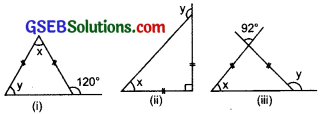Solution:
(i) In the figure, two sides of the triangle are equal.
∴ The base angles opposite to the equal sides are equal.
Since, one of the base angles is y,
∴ Other base angle = y
Now, y and 120° form a linear pair,
∴ y + 120° = 180°
or y = 180° – 120° = 60°
Now, sum of the three angles = 180°
∴ x + y + y =180°
or x + 60° + 60° = 180°
or x + 120° = 180°
or x = 180° – 120° = 60°
Thus, x = 60° and y = 60°.

(ii) In the given figure, two sides of the triangle are equal.
∴ The base angles opposite to equal sides are equal.
Since, one of the base angles is x,
∴ The other base angle = x.
Also, the triangle is a right-angled triangle.
∴ Third angle of the triangle = 90°
Now, sum of the three angles of the triangle = 180°
∴ x + x + 90° = 180°
or 2x + 90° = 180°
or 2x = 180° – 90° = 90°
or $$\frac { 2x }{ 2 }$$ = $$\frac { 90° }{ 2 }$$ or x = 45°
Now, x and y form a linear pair,
∴ x + y =180°
or 45° + y = 180°
or y =180° – 45° = 135°
Thus, x = 45° and y = 135°.

(iii) In the figure, two sides of the triangle are equal.
∴ Base angles are x and x.
The third angle of the triangle = The vertically opposite angle of 92° = 92°
Now, sum of the three angles of the triangle = 180°
∴ x + x + 92° = 180°
or 2x + 92° = 180°
or 2x =180° – 92° = 88°
or $$\frac { 2x }{ 2 }$$ = $$\frac { 88° }{ 2 }$$ or x = 44°
Now, x and y form a linear pair,
∴ x + y = 180°
or 44° + y = 180°
or y = 180° – 44° = 136°
Thus, x = 44° and y = 136°.Think, Discuss and Write (Page 127)

Question 1.
Is the sum of any two angles of a triangle always greater than the third angle?
Solution:
No, the sum of any two angles of a triangle is not always greater than the third angle.

Try These (Page 129)

Question 1.
Find the unknown length x in the following figures.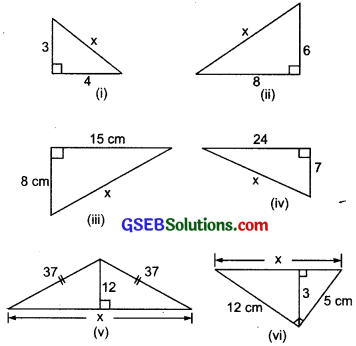Solution:
(i) In the given right triangle, the longest side (hypotenuse) = x.
∴ 3² + 4² = x².
[Using Pythagoras property]
or 9 + 16 = x²
or 25 = x²
x² = 5² ⇒ x = 5

(ii) The given figure is a right-angled triangle.
∴ Using the Pythagoras property, we have x² = 6² + 8²
or x² = 36 + 64 = 100
x² = 10² ⇒ x = 10

(iii) The given figure is a right-angled triangle.
∴ Using the Pythagoras property, we have
x² = 8² + 15²
or x² = 64 + 225 = 289
or x² = 17² ⇒ x = 17 cm

(iv) Using the Pythagoras property in the given right triangle, we have
x² = 7² + 24²
or x² =49 + 576 = 625
x² = 25² ⇒ x = 25

(v) The given figure can be labelled as:In right ∆-I, using the Pythagoras property, we have
y² + 12² = 37²
or y² + 144 = 1369
or y² = 1369 – 144 = 1225
y² = 35² ⇒ y = 35
In right ∆-II, using the Pythagoras property, we have
(x – y)² + 12² = 37²
or (x – 35)² + 144 = 1369
or (x – 35)² = 1369 – 144 = 1225
or (x – 35)² = (35)²
or x – 35 = 35
x = 35 + 35 = 70

(vi) Using the Pythagoras property in rt ∆ABC, we have122² + 5² = x²
or 144 + 25 = x²
or 169 = x²
(13)² = x²
or 13 = x
⇒ x = 13Think, Discuss and Write (Page 131)

Question 1.
Which is the longest side in the triangle PQR, right-angled at P?
Solution:
The vertex containing 90° R is P.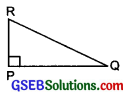∴ Arms of the right angle are PQ and PR
∴ Hypotenuse is QR.
Thus, the longest side is QR.

Question 2.
Which is the longest side in the triangle ABC, right-angled at B?
Solution: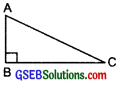∵ The right angle is at B.
∴ The legs of the right triangle are AB and BC.
∴ Hypotenuse is AC.
So, the longest side is AC.

Question 3.
Which is the longest side of a right triangle?
Solution:
In a right triangle, the longest side is its hypotenuse.Question 4.
‘The diagonal of a rectangle produce by itself the same area as produced by its length and breadth ” This is Baudhayan Theorem. Compare it with the Pythagoras property.
Solution:
In the figure, ABCD is a rectangle and BD is its one of the diagonals.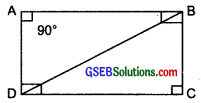According to the Baudhayan Theorem, we have